# DECIMAL Function

Convert a number in a stated base into a decimal value

## What is the DECIMAL Function?

The DECIMAL function in Excel is a Math and Trigonometry function. It is used for converting a text representation of a number in a stated base into a decimal value. The function was introduced in MS Excel 2013.

### Formula

Where:

• Text is the text representation of a number. The text string must be in quotation marks.
• Radix is the base of the number given. It must be an integer and should be greater than or equal to 2 (binary or base2) and less than or equal to 36 (base 36).

If radix is greater than 10, it will use 0-9 numeric values and A-Z letters as required. For example, if we use base 16, it will use 0-9 and A-F. For base 30, it will use 0-9 and A-T.

### How to use the DECIMAL Function in Excel?

To understand how to use this function, let’s consider a few examples:

#### Example 1

Let’s assume we wish to convert the three random letters “C”, “F”, and “A” in base 36 to its equivalent decimal value. The formula to be used would be: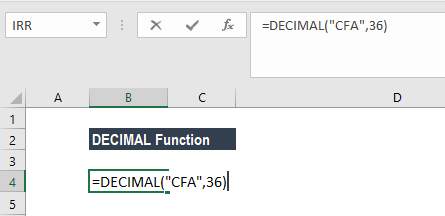This Decimal function used the formula =(12*(36^2))+(15*(36^1))+(10*(36^0)) as “C” is in position 12, “F” in position 15 and “A” in position 10. The result would be: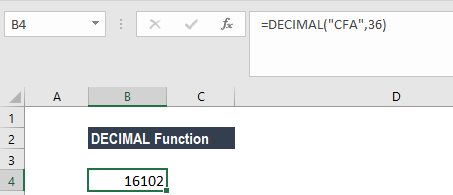#### Example 2

Let’s take this example wherein we wish to convert the binary (base 2) value 1111 to its equivalent decimal.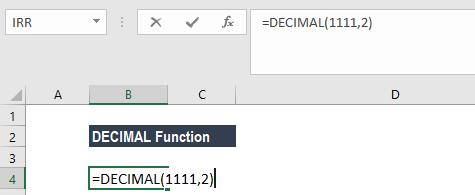The DECIMAL Function will convert 1111 to its equivalent decimal (base10) value of 15.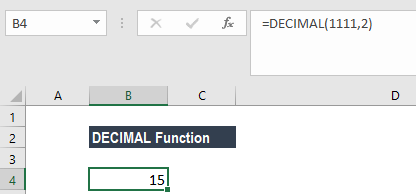Here the formula used is =(1*(2^3)) +(1*(2^2)) +(1*(2^1)) +(1*(2^0)). We can cross check this result with BIN2DEC function. The formula to be used would be BIN2DEC (“1111”).

### Example 3

Let’s assume we wish to convert the hexadecimal (base 16) value FFF to its equivalent decimal (base 10) value (255). The formula that we can use would be: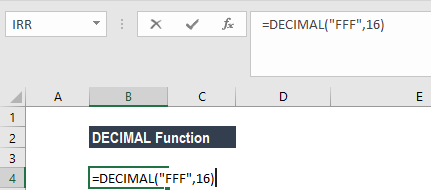The DECIMAL Function used the formula =((15*(16^2)+(15*(16^1))+(15*(16^0)).

Here, F is in position 15 in the base 16 number system. As all number systems start with 0, the 16th character in hexadecimal will be in the 15th position. We would get the result 4095.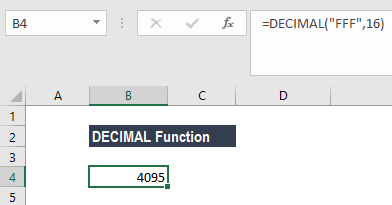#### Example 4

Now, let’s say we wish to convert “I wish to travel” into a decimal.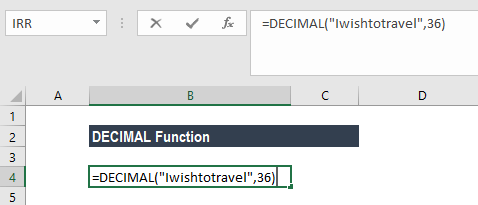The result would be: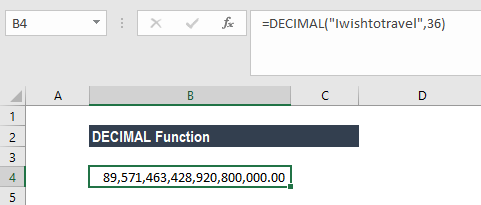#### Example 5

Now, let’s see a few more examples to understand how the DECIMAL function works: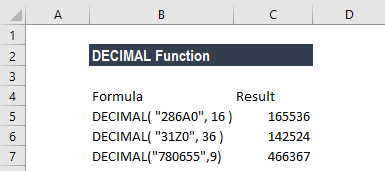### A few pointers about the DECIMAL Function

1. The length of the text must be less than or equal to 255 characters. If the length exceeds 255 characters, the formula gives an error.
2. #NUM! error would occur when the argument is outside its constraints.
• The radix given is < 2 or > 36.
• The text argument given is not recognized as a number in the specified base (radix). For example, if we give the argument =DECIMAL (“200”,2). As 200 is not a valid representation of a binary number, we would get a #NUM! error.
• When the number of characters exceeds 255.
3. #VALUE! error occurs when the text argument or radix is outside its constraints
• When the given text argument is more than 255 characters.
• When the radix given is non-numeric.
4. The radix should be either greater than or equal to 2 (binary or base 2). Also, it should be less than or equal to 36 (base 36). If the given radix is greater than 10, the function will use numeric values (0-9) and the letters A-Z as needed. For example, base 17 would use 0-9 and A-G whereas base 36 would use 0-9 and A-Z.
5. Text argument is not case sensitive so it will provide the same results for =DECIMAL(“atfd”, 30) and =DECIMAL(“ATFD”, 30).

Thanks for reading CFI’s guide to important Excel functions! By taking the time to learn and master these functions, you’ll significantly speed up your financial analysis. To learn more, check out these additional CFI resources:

• Excel Functions for Finance